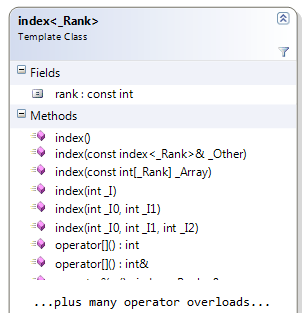## concurrency::index from amp.h

Sun, September 4, 2011, 09:40 PM under GPGPU | ParallelComputing

#### Overview

C++ AMP introduces a new template class index, where N can be any value greater than zero, that represents a unique point in N-dimensional space, e.g. if N=2 then an index<2> object represents a point in 2-dimensional space. This class is essentially a coordinate vector of N integers representing a position in space relative to the origin of that space. It is ordered from most-significant to least-significant (so, if the 2-dimensional space is rows and columns, the first component represents the rows). The underlying type is a signed 32-bit integer, and component values can be negative.

The rank field returns N.

#### Creating an indexThe default parameterless constructor returns an index with each dimension set to zero, e.g.

`  index<3> idx; //represents point (0,0,0)`

An index can also be created from another index through the copy constructor or assignment, e.g.

`  index<3> idx2(idx); //or index<3> idx2 = idx;`

To create an index representing something other than 0, you call its constructor as per the following 4-dimensional example:

```  int temp = {2,4,-2,0};
index<4> idx(temp);```

Note that there are convenience constructors (that don’t require an array argument) for creating index objects of rank 1, 2, and 3, since those are the most common dimensions used, e.g.

```  index<1> idx(3);
index<2> idx(3, 6);
index<3> idx(3, 6, 12);```

#### Accessing the component values

You can access each component using the familiar subscript operator, e.g.

One-dimensional example:

```  index<1> idx(4);
int i = idx; // i=4```

Two-dimensional example:

```  index<2> idx(4,5);
int i = idx; // i=4
int j = idx; // j=5```

Three-dimensional example:

```  index<3> idx(4,5,6);
int i = idx; // i=4
int j = idx; // j=5
int k = idx; // k=6```

#### Basic operations

Once you have your multi-dimensional point represented in the index, you can now treat it as a single entity, including performing common operations between it and an integer (through operator overloading): -- (pre- and post- decrement), ++ (pre- and post- increment), %=, *=, /=, +=, -=,%, *, /, +, -. There are also operator overloads for operations between index objects, i.e. ==, !=, +=, -=, +, –.

Here is an example (where no assertions are broken):

```  index<2> idx_a;
index<2> idx_b(0, 0);
index<2> idx_c(6, 9);
_ASSERT(idx_a.rank == 2);
_ASSERT(idx_a == idx_b);
_ASSERT(idx_a != idx_c);

idx_a += 5;
idx_a += 3;
idx_a++;
_ASSERT(idx_a != idx_b);
_ASSERT(idx_a == idx_c);

idx_b = idx_b + 10;
idx_b -= index<2>(4, 1);
_ASSERT(idx_a == idx_b);```

#### Usage

You'll most commonly use index<N> objects to index into data types that we'll cover in future posts (namely array and array_view). Also when we look at the new parallel_for_each function we'll see that an index<N> object is the single parameter to the lambda, representing the (multi-dimensional) thread index…

In the next post we'll go beyond being able to represent an N-dimensional point in space, and we'll see how to define the N-dimensional space itself through the extent<N> class.